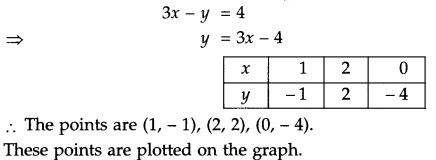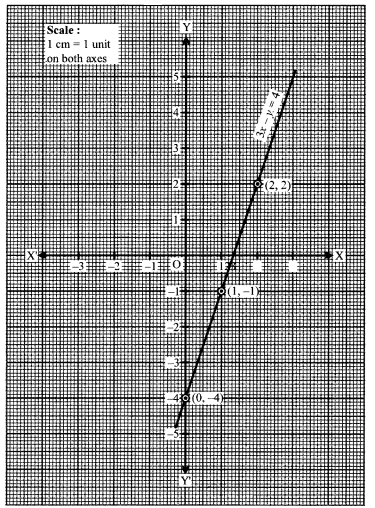ICSE Class 9 Maths Sample Question Paper 4 with Answers

Section – A
(Attempt all questions from this Section)

Question 1.
(a) Expand : $$\left(\frac{2}{3} x-\frac{3}{2 x}-1\right)^{2}$$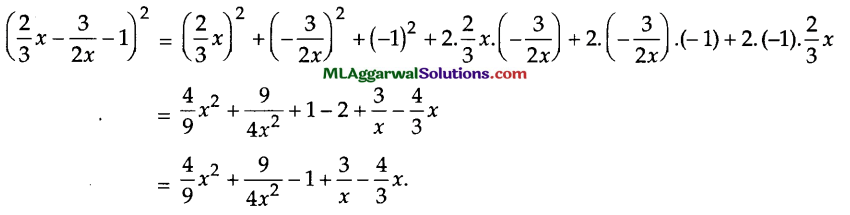(b) A person invests ₹ 10,000 for two years at a certain rate of interest, compounded annu­ally. At the end of one year, this sum amounts to ₹ 11,200. Calculate :
(i) The rate of interest p. a.
(ii) The amount at the end of second year.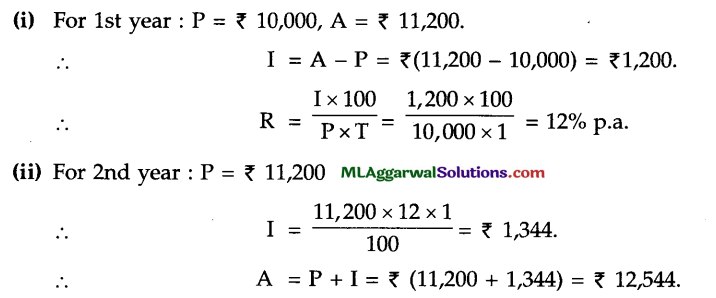(c) Factorize : 64x6 – 729y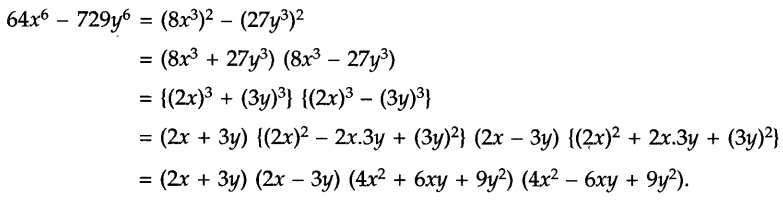Question 2.
(a) If a2 + b2 = 7ab, prove that 2 log (a + b) = log 9 + log a + log b.
Given : a2 + b2 .= 7ab
⇒ a2 + b2 – 9ab – 2ab
⇒ a2 + b2 + 2ab = 9 ab
⇒ (a + b)2 = 9 ab
Taking log of both sides, we get
log (a + b)2 = log (9ab)
⇒ 2 log (a + b) = log 9 + log a + log b.

(b) Prove that: $$\tan ^{2} \theta-\frac{1}{\cos ^{2} \theta}+1=0$$
To prove:
$$\tan ^{2} \theta-\frac{1}{\cos ^{2} \theta}+1=0$$
Consider L.H.S. = $$\tan ^{2} \theta-\frac{1}{\cos ^{2} \theta}+1$$
= tan2 θ- sec2 θ +1
= (1 + tan2 θ) – sec2 θ (∵ 1 + tan2 0 = sec2 0)
= sec2 θ – sec2 θ = θ= R.H.S.
Hence Proved(c) In the given figure, two chords AB and CD of a circle intersect at P. If AB = CD, prove that arc AD = arc CB.
AB = CD
Minor arc AB = Minor arc CD
Minor arc AB – minor arc BD = Minor arc CD – Minor arc BD
⇒ arc AD = arc CB.
Hence Proved.

Question 3.
(a) In the given figure, ∠ BCD = ∠ADC and ∠BCA = ∠ADB.
Show that: (i)ΔACD ≅ ΔBDC (ii) BC = AD (iii) ∠A = ∠B.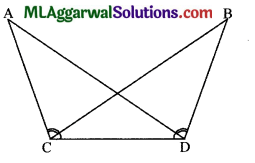=> ∠BCA + ∠BCD = ∠ADB + ∠BCD
=> ∠ACD = ∠BDC
In ΔACD and ΔBDC
CD = CD (Common side)
⇒ ∠ACD = ∠BDC (Proved above)
∴ ΔACD ≅ ΔBDC (ASA axiom)
(iii) ∴ ∠A = ∠B. (c.p.c.t.)
Hence Proved.(b) $$a=\frac{2-\sqrt{5}}{2+\sqrt{5}} \text { and } b=\frac{2+\sqrt{5}}{2-\sqrt{5}}, \text { find } a^{2}-b^{2}$$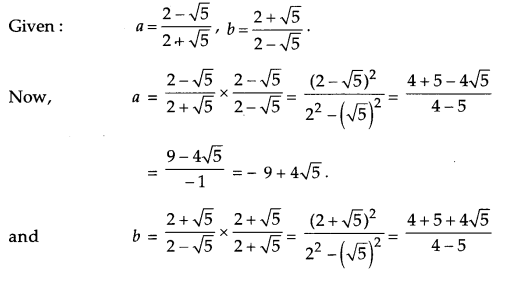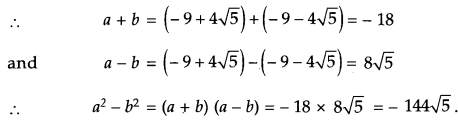(c) Prove that the points A (2, 3), B (-2, 2), C (-1, -2) and D (3, – 1) are the vertices of a square ABCD.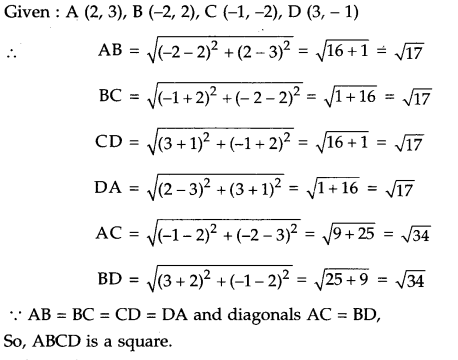Question 4.
(a) The mean height of 10 girls in a class is 1.38 m and the mean height of 40 boys is 1.44 m. Find the mean height of 50 students of the class.
Given : Mean height of 10 girls = 1.38 m
∴ Sum of heights of 10 girls = 1.38 x 10 = 13.8 m
and Mean height of 40 boys = 1.44 m
∴ Sum of heights of 40 boys = 1.44 x 40 = 57.6 m.
∴ Sum of heights of 50 students = 13.8 +57.6 = 71.4 m.
∴ Mean heights of 50 students $$\frac{71.4}{50}$$
=1.428m

(b) In the given figure, AABC is a right triangle with ∠C = 90° and D is mid-point of side BC. Prove that AB2 = 4AD2 – 3AC2.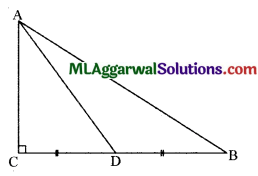Given ∠C = 90°, D is the mid-point of BC.
∴ CD = BD
∴  In ΔABC,
AB2 = AC2 + BC(Pythagoras theorem)
= AC2 + (2CD)2 (∵ CD = BD = $$\frac{1}{2}$$ BC)
AB2 = AC2 + 4 CD2  …(i)
In ΔACD,
AD2 = AC2 + CD2  (Pythagoras theorem)
⇒ CD2 = AD2 – AC2 …(ii)
From equations (i) and (ii), we get
AB2 = AC2 + 4 (AD2 – AC2)
= AC2 + 4AD2 – 4AC2
Hence Proved.(c) The following observation have been arranged in ascending order.
3, 6, 7, 10, x, x + 4, 19, 20, 25, 28 . If the median of the data is 13, find the value of x.
Given : Numbers in ascending order : 3, 6, 7, 10, x, x + 4, 19, 20, 25, 28.
Median = 13
Here, n = 20.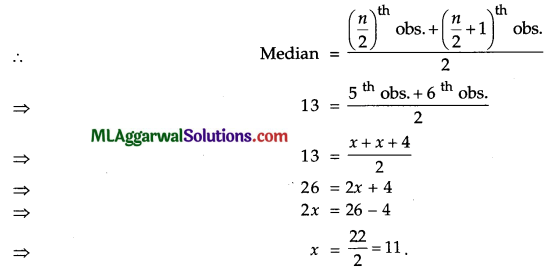Section – B
(Attempt any four questions from this Section)

Question 5.
(a) Factorize : 5x2 + 17xy – 12y2.
5x2 + 17xy – 12y2 = 5x2 + (20 – 3) xy – 12y2
= 5x2 + 20xy – 3xy – 12y2
= 5x(x + 4y) – 3y (x + 4y)
= (x + 4y) (5x – 3y).

(b) If twice the son’s age in years is added to the father’s age, the sum is 70. But if twice the  father’s age is added to the son’s age, the sum is 95. Find the ages of father and son.
Let father’s age be x years and that of son’s be y years.
By 1st condition, x + 2y = 70 …(i)
By 2nd condition, 2x + y = 95 …(ii)
Multiplying equation (ii) by 2, we get
4x + 2y = 190
Subtracting equation (iii) from equation (i), we get⇒ 40 +2y = 70
⇒ 2y = 70 – 40
⇒ $$y=\frac{30}{2}=15$$
∴ Father’s age is 40 years and son’s age is 15 years.(c) In a ΔABC, E and F are the mid-points of AC and AB respectively. The altitude AP to BC intersects FE at Q. Prove that AQ = QP.
Given: E, F are mid-points of sides AC and AB, respectively.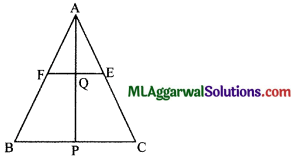We know, the line joining the mid-points of any two sides of a triangle is parallel to the third side
FE || BC
⇒ FQ || BP
Now, since F is mid-point of AB and FQ || BP
∴ By converse of mid-point theorem,
⇒ Q is the mid-point of AP.
AQ = QP. Hence Proved.

Question 6.
(a) If a + $$\frac{1}{a} = p,$$ prove that $$a^{3}+\frac{1}{a^{3}}=p\left(p^{2}-3\right).$$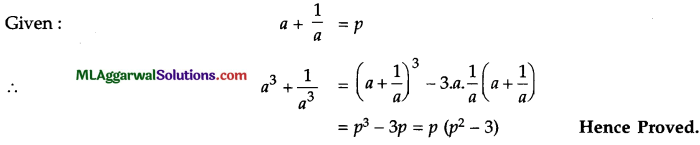(b) The side of a square exceeds the side of another square by 3 cm and the sum of the areas of the two squares is 549 cm2. Find the perimeters of the squares.
Let the side of one square be x cm
Then, side of other square = (x + 3) cm.
Area of two squares are x2 cm2 and (x + 3)2 cm2, respectively.
According to the question,
x2 + (x + 3)2 = 549 ⇒ x2 + x2 + 6x + 9 = 549
⇒ 2x2 + 6x – 540 = 0
⇒ x2 + 3x – 270 = 0
⇒ x2 + (18 – 15)x – 270 =0
⇒ x2 + 15x – 15x – 270 =0
⇒ x (x + 18) – 15 (x + 18) =0
⇒ (x + 18) (x – 15) =0
⇒ x = – 18 or 15
∴ x – 15      (∵ x cannot be negative)
∴ x+ 3 =15+ 3 = 18
∴ Perimeter of one square = 4 x 15 = 60 cm
and Perimeter of other square = 4 x 18 = 72 cm(c) Simplify :
$$\frac{\cos \theta}{\sin \left(90^{\circ}-\theta\right)}+\frac{\cos \left(90^{\circ}-\theta\right)}{\sec \left(90^{\circ}-\theta\right)}-3 \tan ^{2} 30^{\circ}$$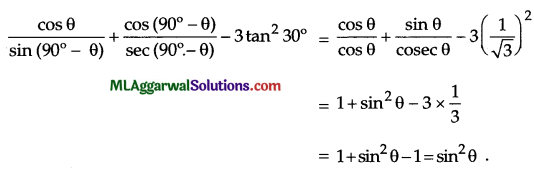Question 7.
(a) If log10 a = b, express 102b3 in terms of a.(b) ΔABC and ΔDBC are on the same base BC with A, D on opposite sides of BC. If area of ΔABC = area of ΔDBC, prove that BC bisects AD.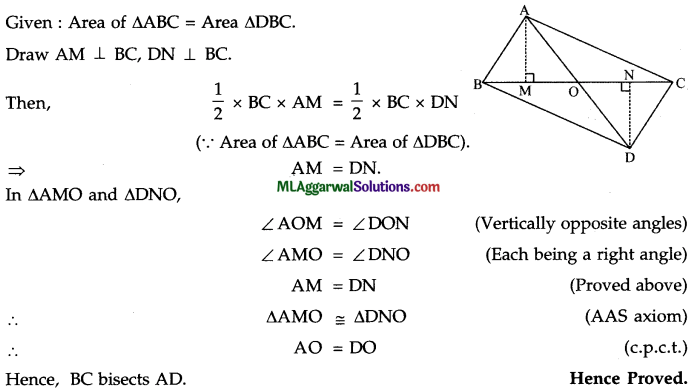(c) If cos θ + sec θ = 2, show that cos8 θ + sec8 θ = 2.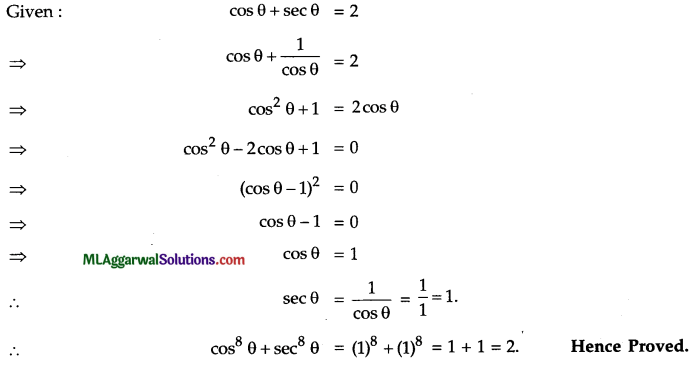Question 8.
(a) If each interior angle is double the exterior angle, find the number of sides.(b) Solve by the substitution method :
5x + 4y – 4 = 0; x – 20 = 12y.
Given: 5x+4y – 4 =0 ………..(i)
and x – 20 =12y ………… (2)
From (ii), x = 12y + 20 ……. (3)
Putting x = 12 + 20 in equation (i), we have
5(12y+20)+4y – 4 =0
⇒ 60y+100+4y – 4=0
⇒ 64y = – 96
⇒ $$y=-\frac{96}{64}=-\frac{3}{2}$$
From (iii), x = 12 x $$\left(\frac{-3}{2}\right)$$ +20 = 2
x = 2,y = $$\frac{-3}{2}$$(c) If x = 2 + √3 , find the value of x – $$\frac{1}{x}$$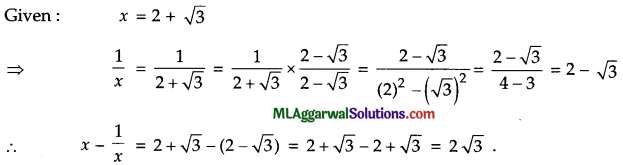Question 9.
(a) Evaluate : $$x^{2 / 3} \cdot y^{-1} \cdot z^{1 / 2}$$ when x – 8, y = 4 and z = 25(b) Construct a ΔABC is which base AB 5 cm, ∠A = 30° and AC – BC – 2.5 cm.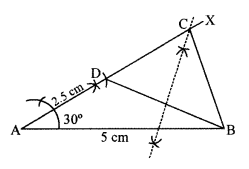Steps of construction :
(1) Draw base AB = 5 cm.
(2) Draw ∠BAX = 30°
(3) From AX, cut-off AD = 2.5 cm
(4) Join BD.
(5) Draw the perpendicular bisector of BD to cut AX at C.
(6) Join BC.
Thus, ABC in the required triangle.

(c) Simplify: $$\left(2 x-\frac{1}{2 x}\right)^{2}-\left(2 x+\frac{1}{2 x}\right)\left(2 x-\frac{1}{2 x}\right)$$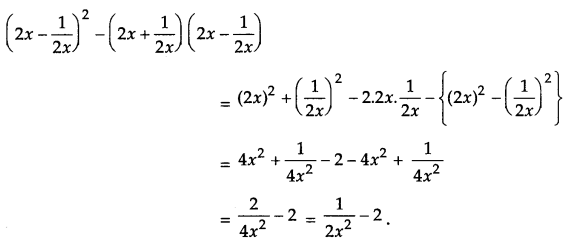Question 10.
(a) Simplify: $$\left(a^{m-n}\right)^{m+n} \cdot\left(a^{n-l}\right)^{n+l} \cdot\left(a^{l-m}\right)^{l+m}$$(b) If area of a semi-circular region is 1232 cm2, find its perimeter.(c) If x = 15°, evaluate : 8 sin cos 4x. sin 6x.
Given: x=15°
∴ 8 sin 2xcos 4x.sin 6x = 8 sin (2 x 15°). cos (4 x 15°) . sin (6 x 15°)
= 8 sin 30° . cos 60° . sin 90°
$$=8 \times \frac{1}{2} \times \frac{1}{2} \times 1=2$$Question 11.
(a) A farmer increases his output of wheat in his farm every year by 8%. This year he pro­duced 2187 quintals of wheat. What was his yearly produce of wheat 2 years ago?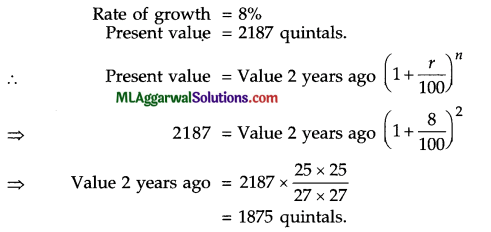(b) Draw the graph of the equation 3x – y = 4.